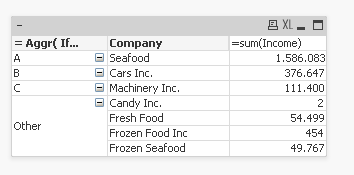Announcements
MAINTENANCE ALERT: Dec. 12th starting 9 AM CET. Community will be read-only. GET DETAILS
cancel
Showing results for
Did you mean:Anonymous
Not applicable

## AGGR Function

Hi, QlikView community.

I have a problem that I have been trying to fix but can't find the solution.

What I am trying to do is make a ABC Groups for my company list. I want to use my last year Revenue (called Income in the dataset) to set my companies to ABC analysis. I have that data below. I have companies names and income for 2016 and 2017. I want to use the income last year to make the rules for what companies are in what groups

 Company Year Income Seafood 2016 1.532.545,78 Cars Inc. 2016 374.290,77 Machinery Inc. 2016 108.202,71 Frozen Seafood 2016 48.767,02 Fresh Food 2016 48.274,13 Frozen Food Inc 2016 0,00 Seafood 2017 53.537 Cars Inc. 2017 2.357 Machinery Inc. 2017 3.198 Frozen Seafood 2017 1.000 Fresh Food 2017 6.225 Frozen Food Inc 2017 454 Candy Inc. 2017 2

So I used this formula below in the calculated dimension. I want to make in the calculated dimension not in script. The problem I face is the company Candy Inc. That company has no income in 2016 and is not put in group

Aggr ( If((sum({1<Year ={'2016'}>}Income) / sum(TOTAL {1<Year ={'2016'}>}Income))> 0.70, 'A',

If((sum({1<Year ={'2016'}>}Income)/ sum(TOTAL {1<Year ={'2016'}>}Income))> 0.15, 'B ',

If((sum({1<Year ={'2016'}>}Income)/ sum(TOTAL {1<Year ={'2016'}>}Income))> 0.05, 'C',

'Other'))),[Company])

So what can I do to get Candy Inc in group Other even though it has no income in the last year?

Any suggestions?

1 Solution

Accepted SolutionsMVP

Try this:

= Aggr( If((sum({1<Year ={'2016'}>}Income)

/ sum(TOTAL {1<Year ={'2016'}>}Income))

> 0.70, 'A',

If((sum({1<Year ={'2016'}>}Income)

/ sum(TOTAL {1<Year ={'2016'}>}Income))

> 0.15, 'B ',

If((sum({1<Year ={'2016'}>}Income)

/ sum(TOTAL {1<Year ={'2016'}>}Income))

> 0.05, 'C',

If(Sum({1} Income) > 0, 'Other',

'Other')))),[Company])2 RepliesMVP

Try this:

= Aggr( If((sum({1<Year ={'2016'}>}Income)

/ sum(TOTAL {1<Year ={'2016'}>}Income))

> 0.70, 'A',

If((sum({1<Year ={'2016'}>}Income)

/ sum(TOTAL {1<Year ={'2016'}>}Income))

> 0.15, 'B ',

If((sum({1<Year ={'2016'}>}Income)

/ sum(TOTAL {1<Year ={'2016'}>}Income))

> 0.05, 'C',

If(Sum({1} Income) > 0, 'Other',

'Other')))),[Company])Anonymous
Not applicable
Author

Hi Darri,

Bring null income rows also in 'others' bracket as following formula,

= Aggr( If((sum({1<Year ={'2016'}>}Income)

/ sum(TOTAL {1<Year ={'2016'}>}Income))

> 0.70, 'A',

If((sum({1<Year ={'2016'}>}Income)

/ sum(TOTAL {1<Year ={'2016'}>}Income))

> 0.15, 'B ',

If((sum({1<Year ={'2016'}>}Income)

/ sum(TOTAL {1<Year ={'2016'}>}Income))

> 0.05, 'C',

If(Sum({1} Income) > 0, 'Other',

'Other')))),[Company])

Please let us know if above is working.

Thanks,

PoojaCommunity Browser